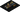# Technology

## Technology

We created a full stack toolchain so you can focus on doing what you love, creating your ML models for your application.

Designed for the developer, we provide the power of our accelerator card with the flexibility of current AI libraries. Accelerate any AI model with 3 lines of code, from image to audio and from fully connected networks to complex GANs.

We provide the technology stack to accelerate your machine learning.## Using the toolchain

Use your favourite library and add just 3 lines of code to accelerate your model. Here are some examples:

## Training

`import torchimport torch.nn as nnimport npu# Preprocess and pack your data...# Create model architecture in Pytorch...# Compile the Pytorch model for the NPUmodel = npu.compile(model,                    input_shape = (3, 224, 224),                    library = 'pytorch')# Train your modelmodel.train(training_data = (x_train, y_train),            test_data = (x_test, y_test),            loss = npu.f.MSELoss,            optim = npu.f.optim.SGD(lr=0.1),            batch_size = 32)# That's it!# Your model just got trained with the NPU`

## Inference

`import torchimport torch.nn as nnimport npu# Preprocess and pack your data...# Load your Pytorch model...# Compile the Pytorch model for the NPUmodel = npu.compile(model,                    input_shape = (3, 224, 224),                    library = 'pytorch')# Run your network with your datapredictions = model.predict(x)# That's it!# Your model just predicted with the NPU`

## Training

`import tensorflow as tfimport npu# Preprocess and pack your data...# Create model architecture in TensorFlow...# Compile the TensorFlow model for the NPUmodel = npu.compile(model,                    input_shape = (3, 224, 224),                    library = 'tensorflow')# Train your modelmodel.train(training_data = (x_train, y_train),            test_data = (x_test, y_test),            loss = npu.f.MSELoss,            optim = npu.f.optim.SGD(lr=0.1),            batch_size = 32)# That's it!# Your model just got trained with the NPU`

## Inference

`import tensorflow as tfimport npu# Preprocess and pack your data...# Load your TensorFlow model...# Compile the TensorFlow model for the NPUmodel = npu.compile(model,                    input_shape = (3, 224, 224),                    library = 'tensorflow')# Run your network with your datapredictions = model.predict(x)# That's it!# Your model just predicted with the NPU`

## Training

`import tensorflow.keras as kimport npu# Preprocess and pack your data...# Create model architecture in Keras...# Compile the Keras model for the NPUmodel = npu.compile(model,                    input_shape = (3, 224, 224),                    library = 'tensorflow-k')# Train your modelmodel.train(training_data = (x_train, y_train),            test_data = (x_test, y_test),            loss = npu.f.MSELoss,            optim = npu.f.optim.SGD(lr=0.1),            batch_size = 32)# That's it!# Your model just got trained with the NPU`

## Inference

`import tensorflow.keras as kimport npu# Preprocess and pack your data...# Load your Keras model...# Compile the Keras model for the NPUmodel = npu.compile(model,                    input_shape = (3, 224, 224),                    library = 'tensorflow-k')# Run your network with your datapredictions = model.predict(x)# That's it!# Your model just predicted with the NPU`

## Your favourite library is not here?

We are always improving our technology, please let us know your suggestions and if this is something you would like to contribute, visit our jobs site or get in touch with us.

## How do we make it fast

Our proprietary Neural Processing Unit, the NPU, has been designed from the ground up to accelerate machine learning workloads by focusing on data movement and processing power. Combined with 128GB of high-speed RAM and many other features, our accelerator card allows your models to fly in the cloud(s).